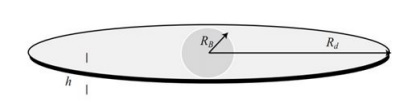# Cosmology - Spiral Galaxy Rotation Curves

In this chapter, we will discuss regarding Spiral Galaxy Rotation Curves and evidence for Dark Matter.

### Dark Matter and Observational Fact about Dark Matter

• The Early Evidence of Dark Matter was the study of the Kinematics of Spiral Galaxy.

• The Sun is offset 30,000 lightyears from the centre of our Galaxy. The galactic centric velocity is 220 km/s.

• Why is the velocity 220 km/s not 100 km/s or 500 km/s? What governs the circular motion of object?

• Mass enclosed within the radius helps to detect the velocity in the Universe.

### Rotation of Milky Way or Spiral Galaxy – Differential Rotation

• Angular Velocity varies with the distance from the centre.

• Orbital time-period depends on the distance from the centre.

• Material closer to Galactic centre has a shorter time-period and material far away from the Galactic Centre has a larger time period.

### Rotation Curve

• Predict the velocity change with the Galactic centric radius. The curve which gives the velocity changes with the orbital radius.

• When we see things move, we think that it is gravity which influences the rotation.

• Mass distribution varies with the radius. Matter density will predict the rotation curve. The rotation curve based on the matter density, which varies with radius.

### Surface Brightness

• We choose the patch and see how much of light is coming out.

• Amount of the light coming from the patch is called as the Surface Brightness.

• Its unit is mag/arcsec2.

• If we find the surface brightness varies with the radius, we can find the luminous matter varies with radius.

$$\mu(r) \propto exp \left( \frac{-r}{h_R} \right )$$

$h_R$ is scale length. $\mu(r) = \mu_o \ast exp \left( \frac{-r}{h_R} \right )$

• $h_R$ is nearly 3 kpc for the Milky way.

## Spiral Galaxies

For the Astronomers to understand the rotational curve, they split the Galaxies into two components, which are −

• Disk
• Bulge

The following image shows a Central spherical bulge + Circular disk. Stellar and gas distribution is different in the bulge and the disk.### Kinematics of Spiral Galaxies

• The Circular velocity of any object – For the bulge is (r < Rb).

$$V^2(r) = G \ast \frac{M(r)}{r}$$

$$M(r) = \frac{4\pi r^3}{3} \ast \rho_b$$

• For the disk – (Rb < r < Rd)

$$V^2(r) = G \ast \frac{M(r)}{r}$$

• Bulge has a roughly constant density of stars.

• Density within the Bulge is constant (not changing with the distance within the bulge).

• In a disk, the stellar density declines with the radius. The radius increases then the luminous matter decrease.

• In Bulk – $V(r) \propto r$

• In Disc – $V(r) \propto 1/\sqrt{r}$

### Rotational Curve of Spiral Galaxies

• Through the Spectroscopy (nearby galaxies – spatially resolved the galaxy), we produce the rotation curve.

• As mentioned above, we see that the rotation curve is flat at the outer regions, i.e. the things are moving fast in the outer regions, which is generally not expected to be in this form.

• The orbital speed increases with the increase in the radius of the inner region, but it flattens in the outer region.

## Dark Matter

The Dark Matter is said to be the Non-Luminous Component of the Universe. Let us understand about dark matter through the following pointers.

• The flat rotation curves are counter to what we see for the distribution of stars and gas in the spiral galaxies.

• The surface luminosity of the disk falls off exponentially with radius, implying that the mass of luminous matter, mostly stars, is concentrated around the galactic center.

• The flattening of the rotation curve suggests that the total mass of the galaxy within some radius r is increasing always with increase in r.

• This can only be explained if there is a large amount of invisible gravitating mass in these galaxies which is not giving out electromagnetic radiation.

• The rotation curve measurements of spiral galaxies is one of the most compelling set of evidences for dark matter.

### Evidence of Dark Matter

• Missing Mass – 10 times the luminous mass.

• Most of this dark matter must be in the halo of the galaxy: Large amounts of dark matter in the disk can disturb the long-term stability of the disk against tidal forces.

• Some small fraction of the dark matter in the disk can be baryonic – dim stars (brown dwarfs, black dwarfs), and compact stellar remnants (neutron stars, black holes). But such baryonic dark matter cannot explain the full scale of missing mass in galaxies.

• Density Profile of Dark Matter – $M(r) \propto r$ and $\rho(r) \propto r^{−2}$.

• The rotation curve data for spiral galaxies are consistent with the dark matter distributed in their halo.

• This dark halo constitutes much of the galaxy’s total mass.

• All baryonic matter (stars, star clusters, ISM, etc.) are held together by the gravitational potential of this dark matter halo.

### Conclusion

• Dark matter has only been detected through their gravitational interaction with an ordinary matter. No interaction with light (no electromagnetic force) has yet been observed.

• Neutrinos − Charge less, weakly interacting, but mass is too less (< 0.23 eV). DM particles should have E > 10 eV or so to explain structure formation.

• Weakly Interacting Massive Particles (WIMPS) can be the source of Dark Matter.

### Points to Remember

• Material closer to Galactic centre has a shorter time-period.

• Bulge has a roughly constant density of stars.

• Surface luminosity of the disk falls off exponentially with radius.

• Large amounts of dark matter in the disk can disturb the long-term stability of the disk against tidal forces.